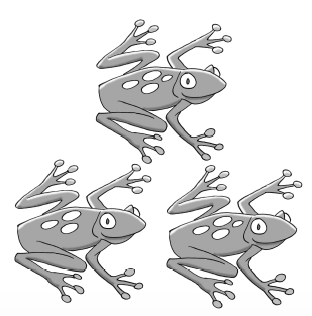### Home > AC > Chapter 3 > Lesson 3.1.7 > Problem3-65

3-65.

ONE OF THESE POINTS IS NOT LIKE THE OTHERS, Part Two

1. Plot and connect the points listed in the table below.

 IN (x) OUT (y) $–2$ $4$ $1$ $−4$ $0$ $3$ $−3$ $2$ $−1$ $0$ $12$ $−3$ $12$ $−4$ $5$ $−2$ $0$ $−3$
2.Identify the point that does not fit the pattern.

Pay attention to how the points look on the graph. Which point does not seem to fit the pattern?

3. What shape does the graph appear to make?

Think of the different types of graphs you have learned.

The graph looks like a parabola.

4. Correct the point identified in part (b) so it fits the pattern. Write the points in $(x,y)$ notation.

Keep the $x$-value the same and find which $y$-value would fit the pattern. Refer to the table and the graph.

$(-3,-2)$ should be $(-3,5)$

Complete the table in the eTool below.
Click the link at right for the full version of the eTool: 3-65 HW eTool (Desmos)